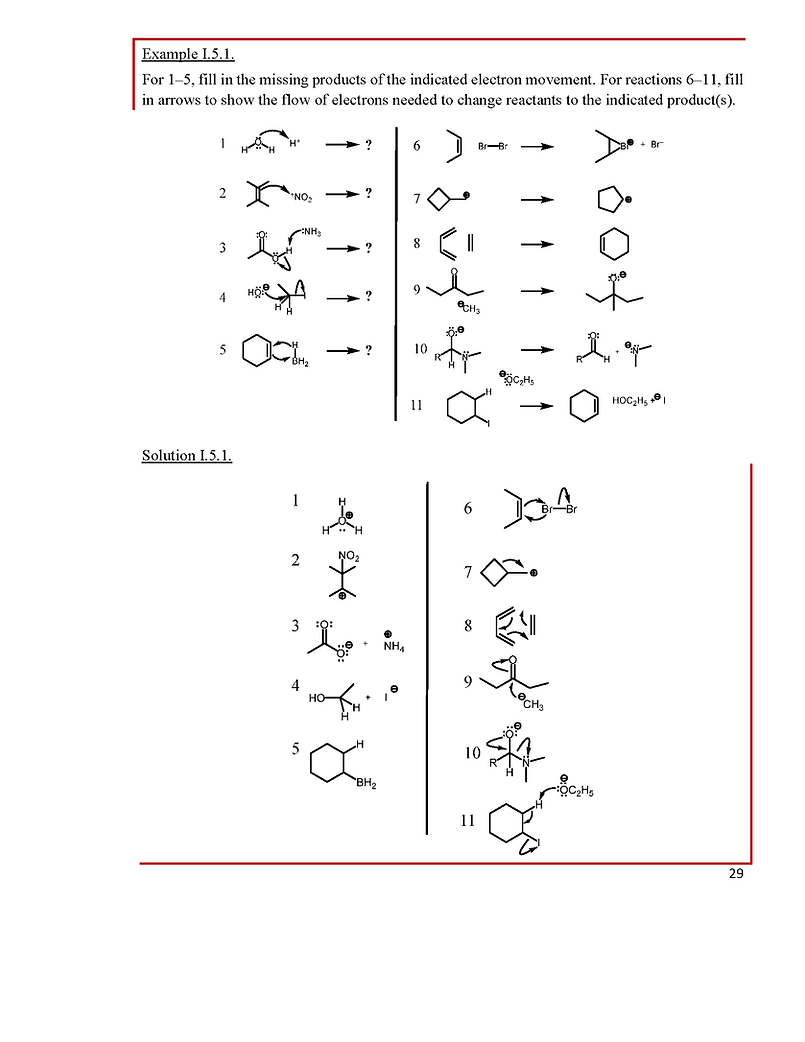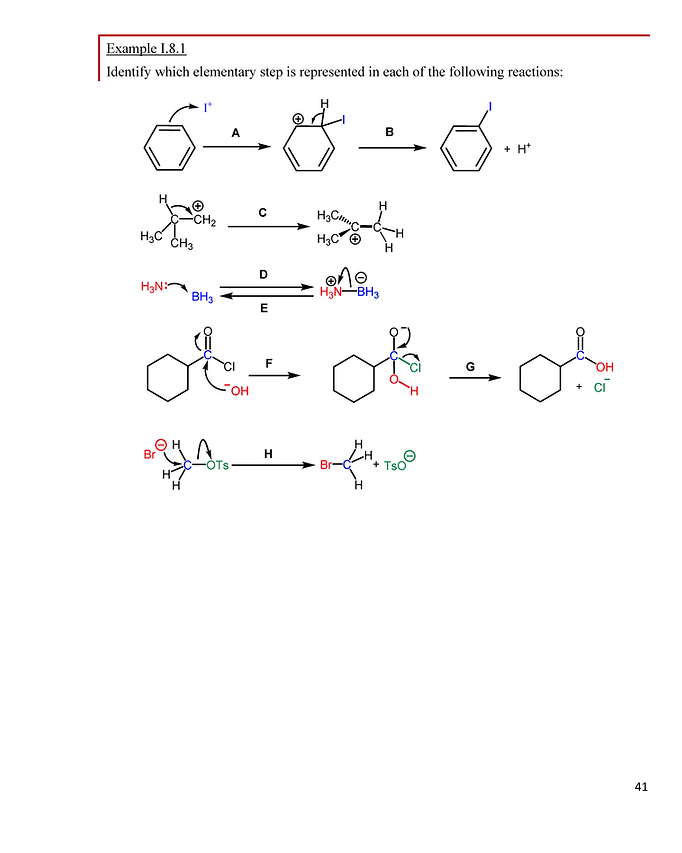### Step 1: Read the Lesson### Step 1: Read the Lesson### Step 1: Read the Lesson### These are free-response questions worked out for you. You will get the most out of these practice problems if you pause the video and try each problem, then watch the solution. This way you will know whether or not you can complete these type of problems yourself.### Step 1: Read the Lesson### These are free-response questions worked out for you. You will get the most out of these practice problems if you pause the video and try each problem, then watch the solution. This way you will know whether or not you can complete these type of problems yourself.### Step 1: Read the Lesson### These are free-response questions worked out for you. You will get the most out of these practice problems if you pause the video and try each problem, then watch the solution. This way you will know whether or not you can complete these type of problems yourself.### Step 1: Read the Lesson### Step 1: Read the Lesson### Step 1: Read the Lesson### Step 1: Read the Lesson### Step 1: Read the Lesson### These are free-response questions worked out for you. You will get the most out of these practice problems if you pause the video and try each problem, then watch the solution. This way you will know whether or not you can complete these type of problems yourself.### Step 1: Read the Lesson### Step 1: Read the Lesson### Step 1: Read the Lesson### These are free-response questions worked out for you. You will get the most out of these practice problems if you pause the video and try each problem, then watch the solution. This way you will know whether or not you can complete these type of problems yourself.### Step 1: Read the Lesson### Step 1: Read the Lesson### Step 1: Read the Lesson### These are free-response questions worked out for you. You will get the most out of these practice problems if you pause the video and try each problem, then watch the solution. This way you will know whether or not you can complete these type of problems yourself.### Step 1: Read the Lesson### The stereochemistry free response practice problem video for this lesson is combined with the one for Lesson I.18. Please do the reading and mini-quiz for Lesson I.18 next!### Step 1: Read the Lesson### Step 1: Read the Lesson### These are free-response questions worked out for you. You will get the most out of these practice problems if you pause the video and try each problem, then watch the solution. This way you will know whether or not you can complete these type of problems yourself.### Step 1: Read the Lesson### These are free-response (first video) AND multiple choice (second video) questions worked out for you. You will get the most out of these practice problems if you pause the video and try each problem, then watch the solution. This way you will know whether or not you can complete these type of problems yourself.